# Einstein's Mass-Energy Equivalence Formula

Einstein's Mass-Energy Equivalence Formula

Albert Einstein's most famous equation shows that mass can be converted to energy, and energy can be converted to mass. This means, in essence, that mass and energy are equivalent concepts. The energy produced by complete conversion of mass to energy is equal to the mass of an object times the speed of light squared. Note that this formula applies to the "rest mass" of an object. For fast-moving objects, special relativity applies, and a different formula is required to find the total energy. The unit of energy is Joules (J), where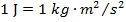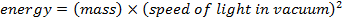E = energy (Joules, J)

m = mass (kg)

c = speed of light in vacuum (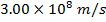)

Einstein's Mass-Energy Equivalence Formula Questions:

1) What is the rest energy of 1kg of water (or any other substance)?

Answer: The rest energy, or the amount of energy that would be released if mass were converted entirely to energy, can be found using Einstein's mass-energy equivalence formula: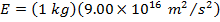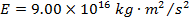The rest energy of 1kg of water, or any other substance, is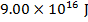. A tremendous amount of energy is associated with the concept of mass.

2) The rest energies of particles are often expressed in units of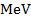, where "" means "mega-electron Volts". An electron Volt is a unit of energy, and can be converted to Joules: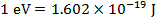. "Mega" refers to "a million", and so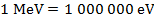. If the rest energy of a neutron is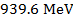, what is the mass of a neutron, in kg?

Answer: The first step to solving the problem is to convert the rest energy of a neutron to Joules. The necessary conversions are given in the question. The rest energy of a neutron is: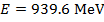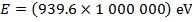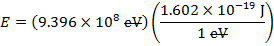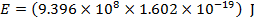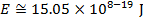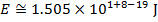The next step is to rearrange Einstein's mass-energy equivalence formula to solve for the mass: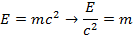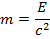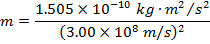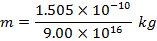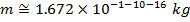The rest mass of a neutron is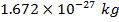.